# Kernel Programming

2023-09-25

List of Figures

1.1. Kernel-Based: Used Page Extent
1.2. Kernel-Based: Applying the Algorithm

List of Examples

1.1. How to Implement a Kernel-Based Module

## Chapter 1. Implementing Kernel-Based Algorithms

Many image filters are based on the so-called kernel-based image filtering. The following section will explain how to implement such filters efficiently.

Main sections are

## 1.1. General Approach

When a fixed region around a voxel is needed to calculate output voxels (edge detector operations, morphological operations, noise filters, smoothing, texture filters, ...) we talk about a kernel-based filter.

Examples are the modules `KernelExample`, `Convolution`, `RankFilter`, `Morphology`.

Figure 1.1. Kernel-Based: Used Page Extent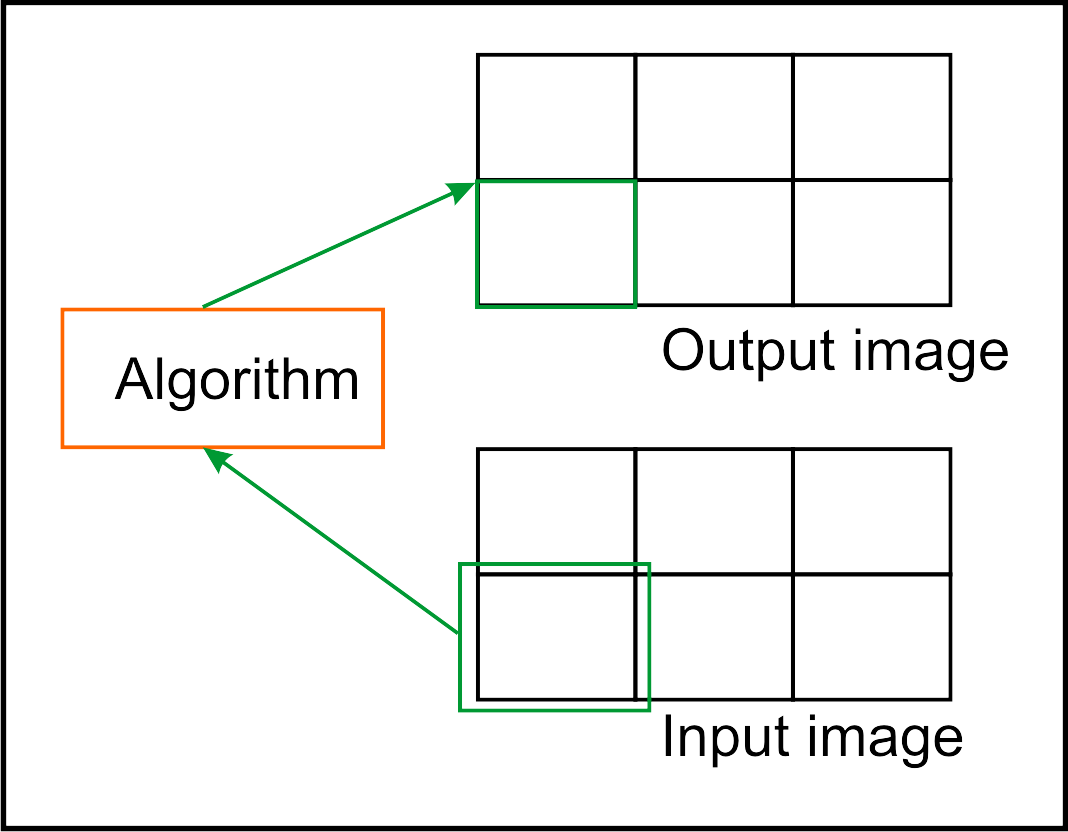Figure 1.2. Kernel-Based: Applying the Algorithm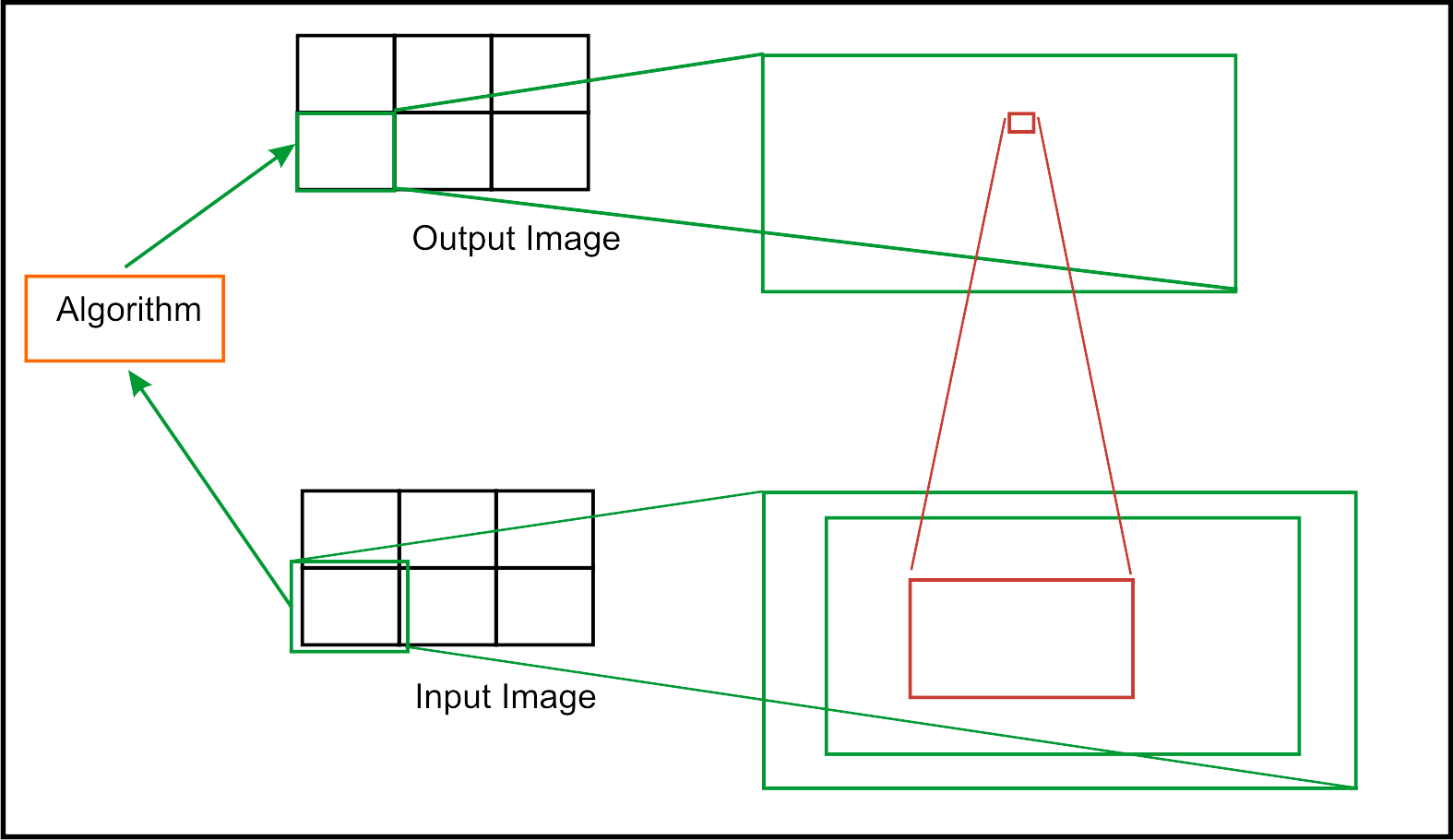• Fast access to kernel range in 6D is possible with paging -> fits well into page concept

• Many algorithm categories can be implemented

• Base class is a bit more complex

• Image borders require consideration (supported by base classes, though)

For a kernel-based image processing approach, some measures must be taken:

• Adjust the extent of output image in `Module::calculateOutputImageProperties()`

• Calculate the extent of input pages in `Module::calculateInputSubImageBox()`

• Apply border handling in `Module::calculateOutputSubImage()`

• Apply kernel to page in `Module::calculateOutputSubImage()`

These measures include many complex steps which are supported by the following classes:

• `KernelBaseModule`

This is a class for page-based kernel operations. Fields for the border handling mode and fill value are automatically created. Macros are available for simple implementation and usage of the kernel algorithm template.

• `KernelModule`

Defines a convenience class for kernel base image filtering. Many convenience methods are available to configure the module with certain field combinations so that derived modules do not have to implement most inputs like kernel extent, fill value, kernel input and output connectors, image and kernel intervals, etc.

• `Kernel`

Manages a kernel matrix with value access, creation, manipulation and (de)coding as a string as well as value copy and assignment, etc.

The class `Kernel` manages a 6D kernel which can be applied to images. It handles a set of coordinates (see `Kernel::getCoordTab()`) and values for those coordinates (see `getValueTab()`). This permits the specification of kernels with gaps or only a very few defined elements. Thus big kernels with only a few elements can be manipulated and applied fast. A set of operations is available on a kernel instance which includes arithmetics on the kernel values, gauss presets, normalization, different kernel set/get routines to create/save partially defined kernels, get/set methods to load/save kernels as strings or arrays, and much more.

This `Kernel` class is implemented as a template dependent on `KDATATYPE` to have different precisions for kernel elements. Usually, the kernel is instantiated with `MLdouble` as `KDATATYPE`. This type is also given as typedef `KernelDataType` which should be used e.g., when pointers to the table of kernel elements are needed. This class can be instantiated with `MLfloat` or `MLdouble` as `KDATATYPE`; however, the Kernel base classes always use `KernelDataType`.

Note that using integer types for the kernels is not really useful since pure integer kernel operations are rare and some operations would suffer because rounding errors occur.

## 1.2. Border Handling in Kernel Operations

For image filtering, image borders must be considered. Some modes are supported by the class `KernelBaseModule` and are automatically applied by the derived kernel filters.

• `KernelTools::NO_PAD`

Only those voxels are passed to the output which can be filtered correctly by the entire kernel. Hence the output image is usually shrinked by the extent of the kernel minus 1.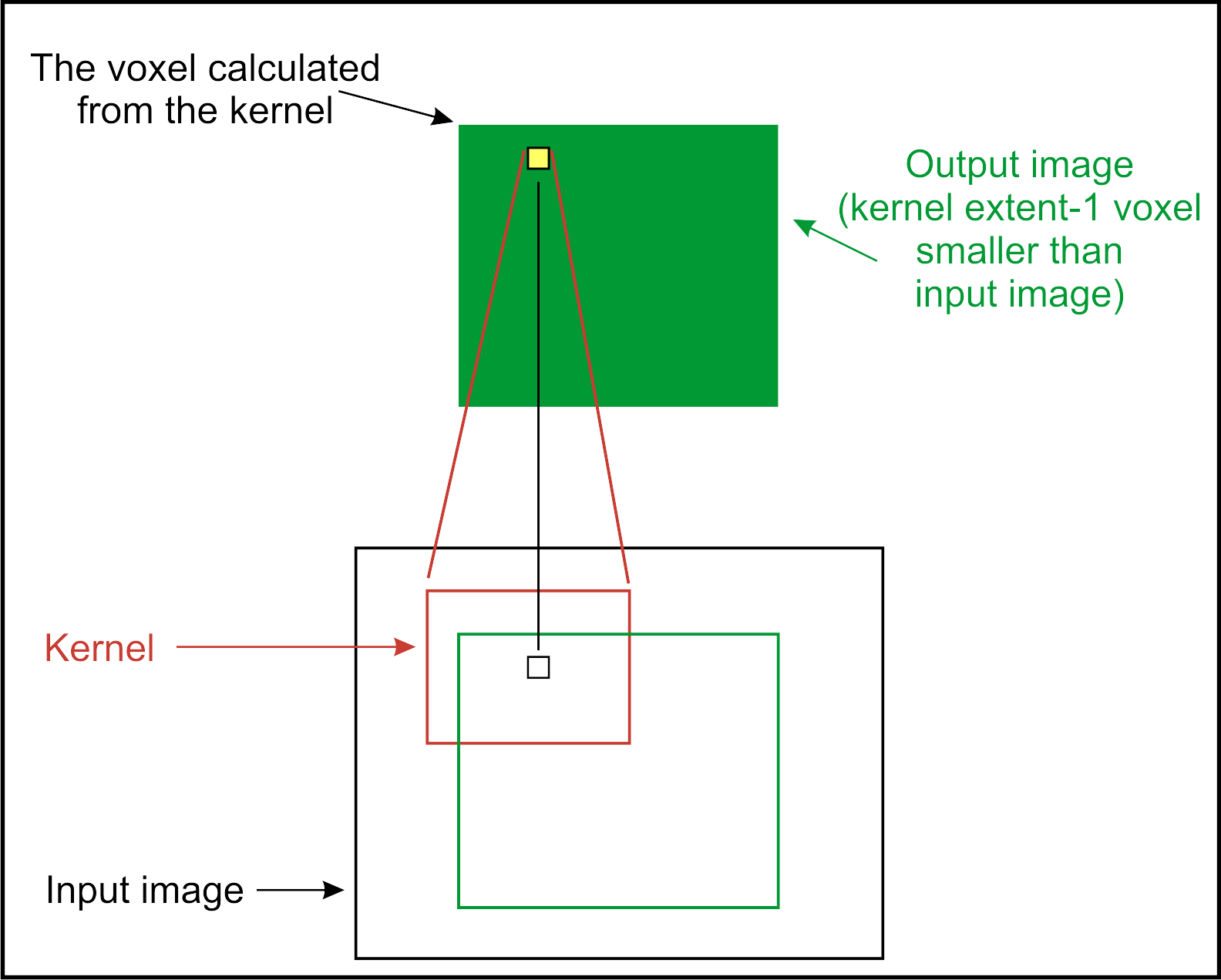• `KernelTools::PAD_SRC_FILL`

The input image is virtually expanded by some voxels so that the kernel can filter all input image voxels correctly. The area added around the input image is filled with the fill value.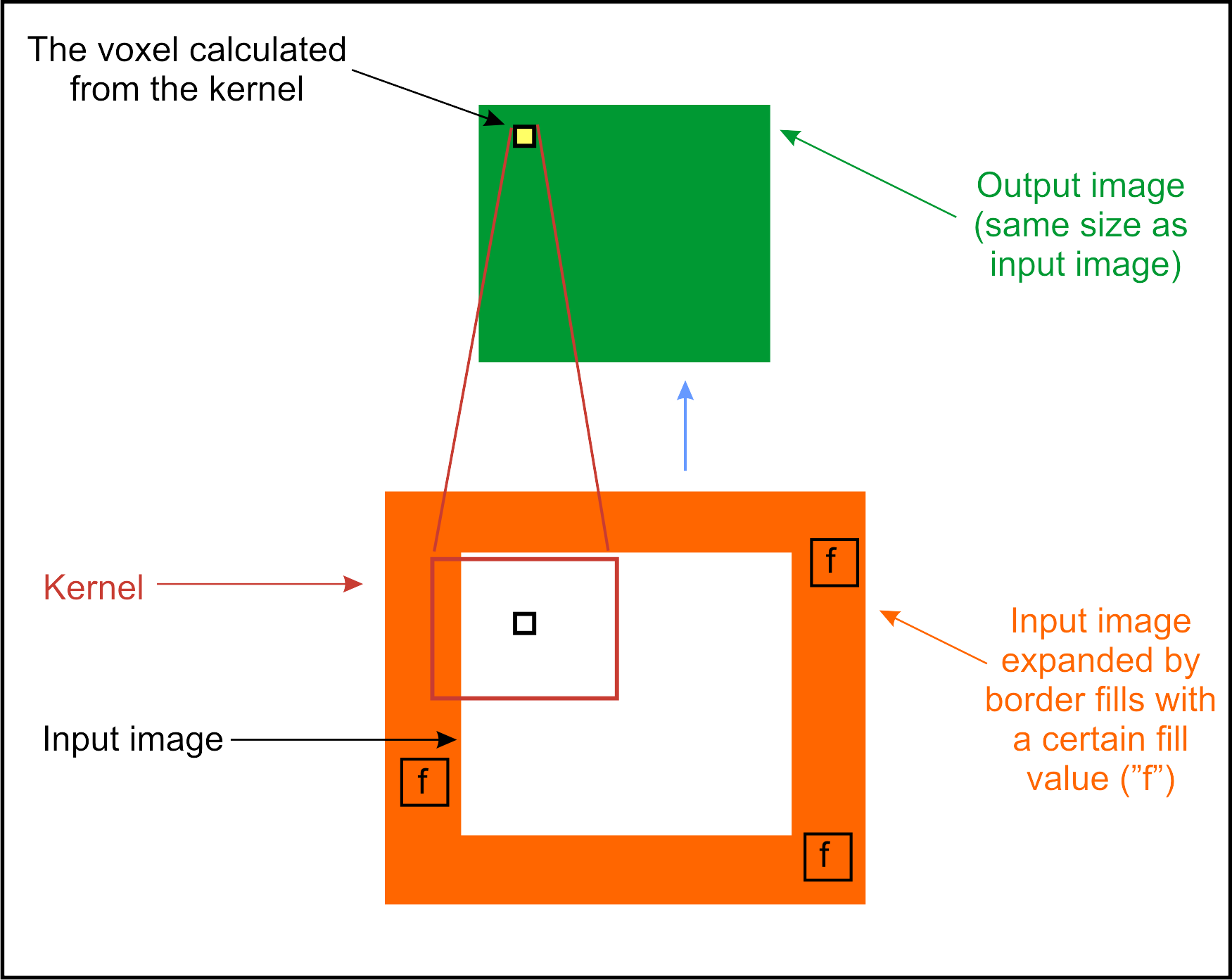• `KernelTools::PAD_DST_FILL`

The kernel filters all pixels of the output image if the kernel can filter them correctly without accessing voxels outside the output image. The remaining voxels of the image are filled with the fill value.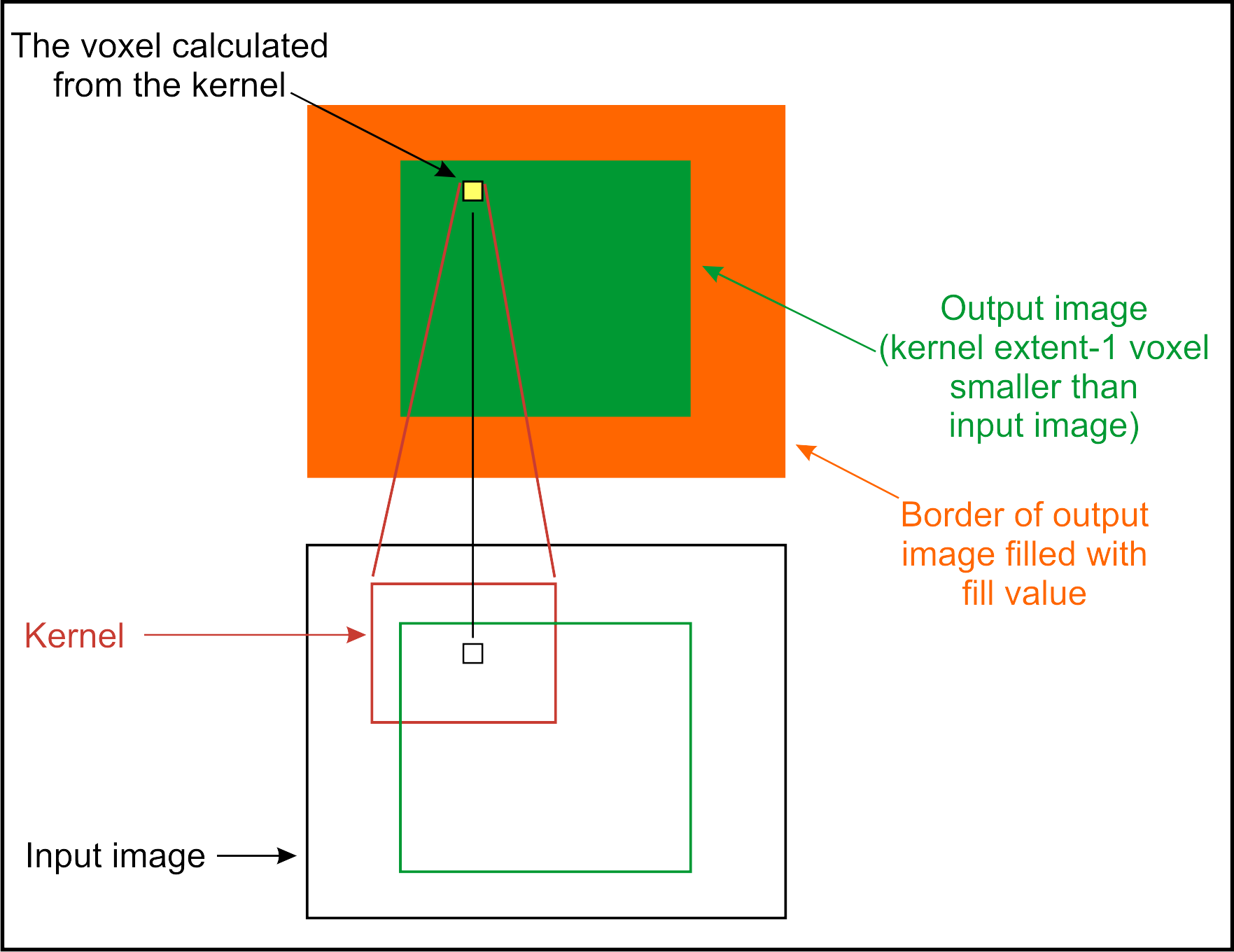• `KernelTools::PAD_DST_ORIGFILL`

The kernel filters all voxels of the output image if the kernel can filter them correctly without accessing voxels outside the output image. All other voxels are copied from the input image.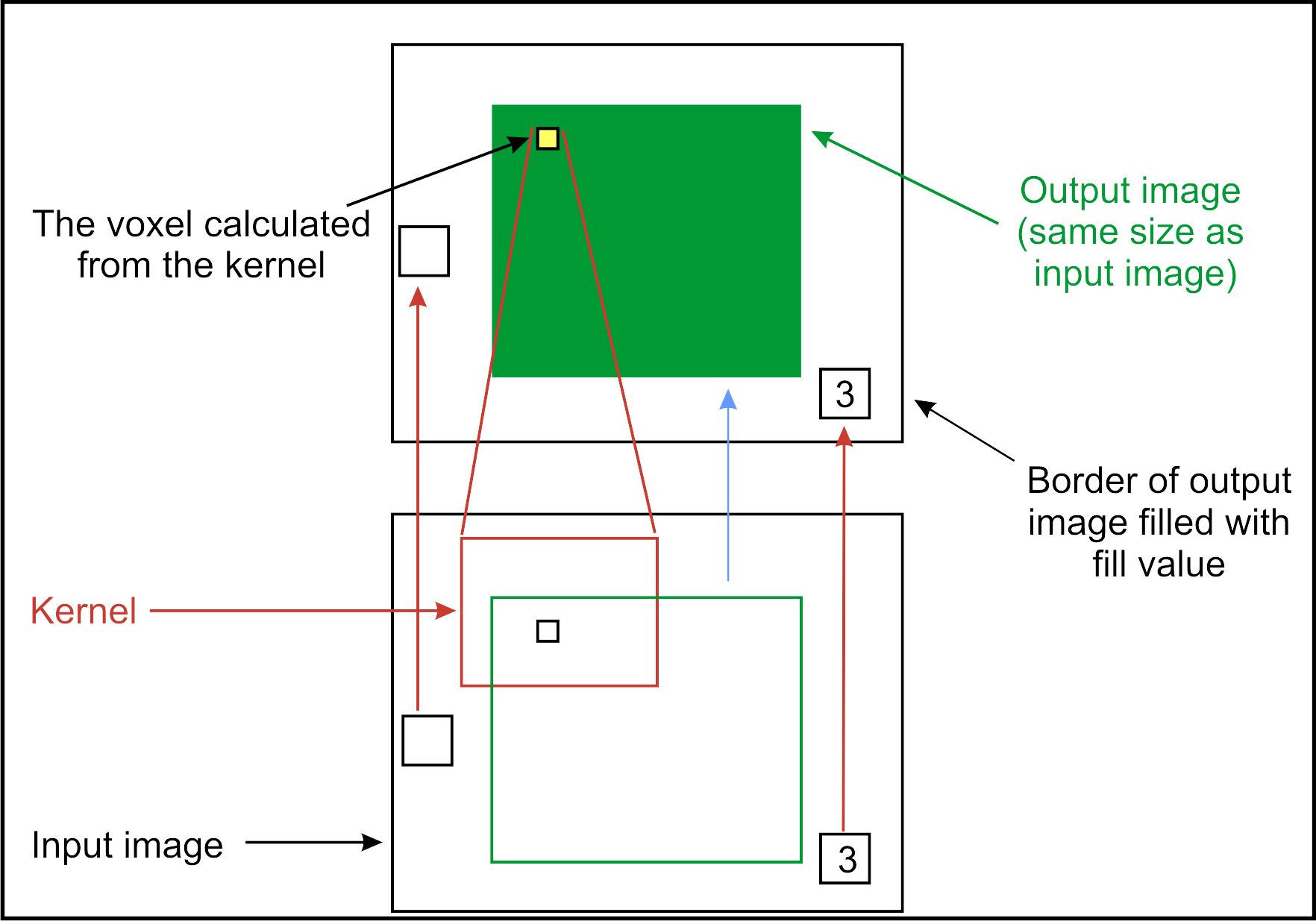• `KernelTools::PAD_SRC_UNDEFINED`

The input image is virtually expanded by some voxels so that the kernel can filter all input image voxels correctly. The contents of the area added around the input image are left undefined. Hence, the filtered image will also have a border with undefined image values.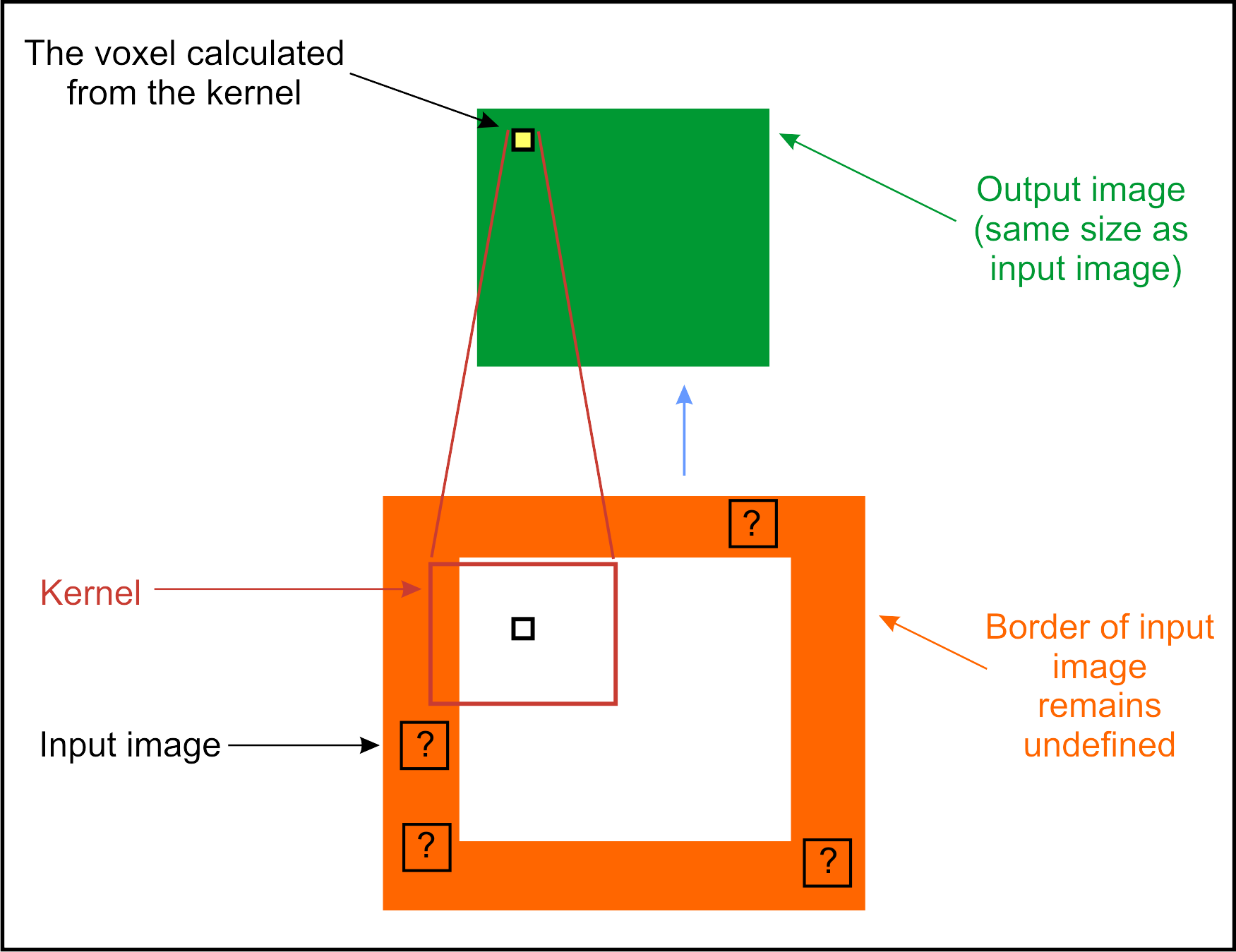• `KernelTools::PAD_DST_UNDEFINED`

The kernel filters all voxels of the output image if the kernel can filter them correctly without accessing voxels outside the output image. All other voxels are left undefined.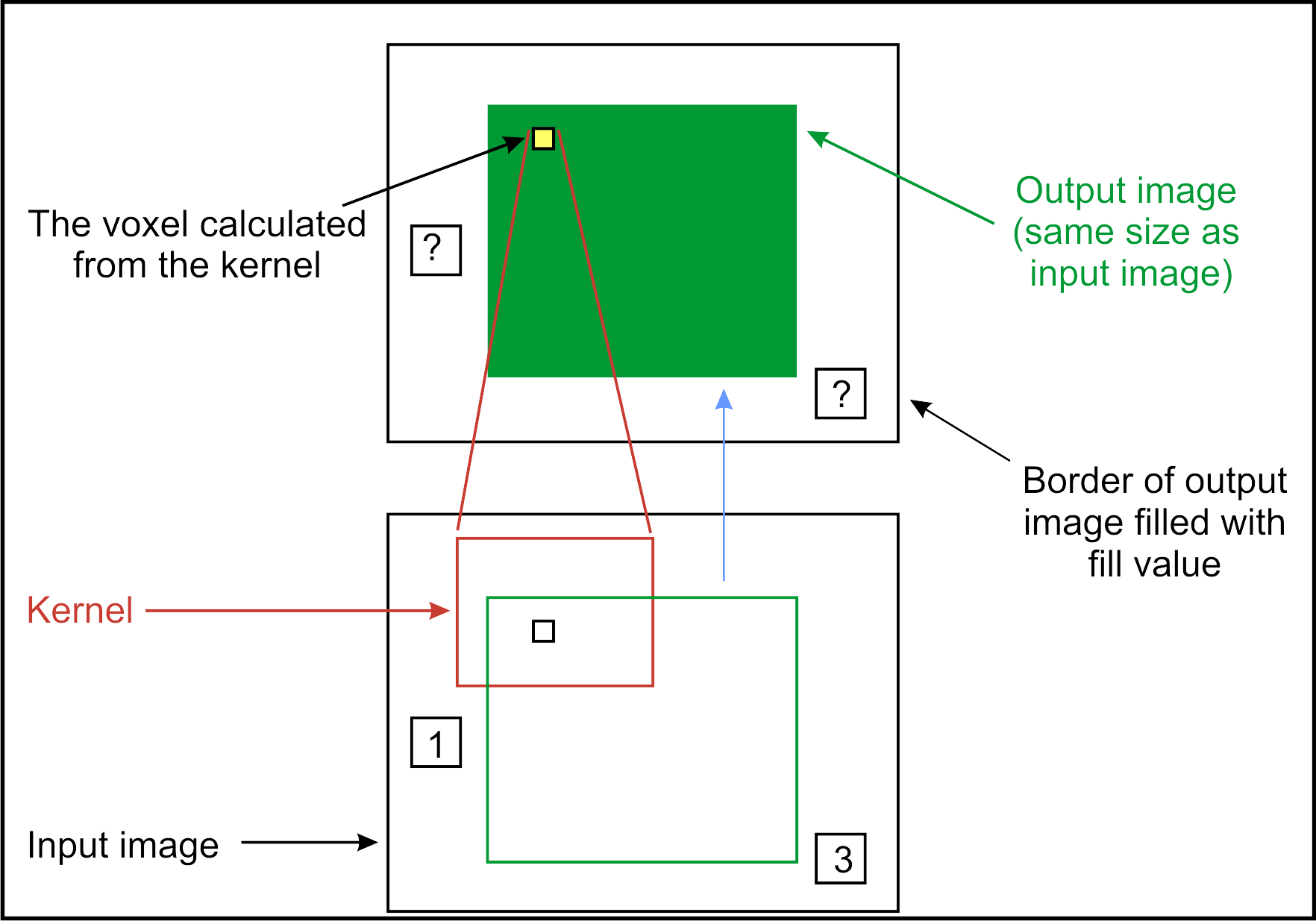• `KernelTools::PAD_SRC_CLAMP`

The input image is virtually expanded by some voxels so that the kernel can filter all input image voxels correctly. The contents of the area added around the input image are filled with the nearest voxel found in the input image. This is usually the mode which produces the best results for image borders.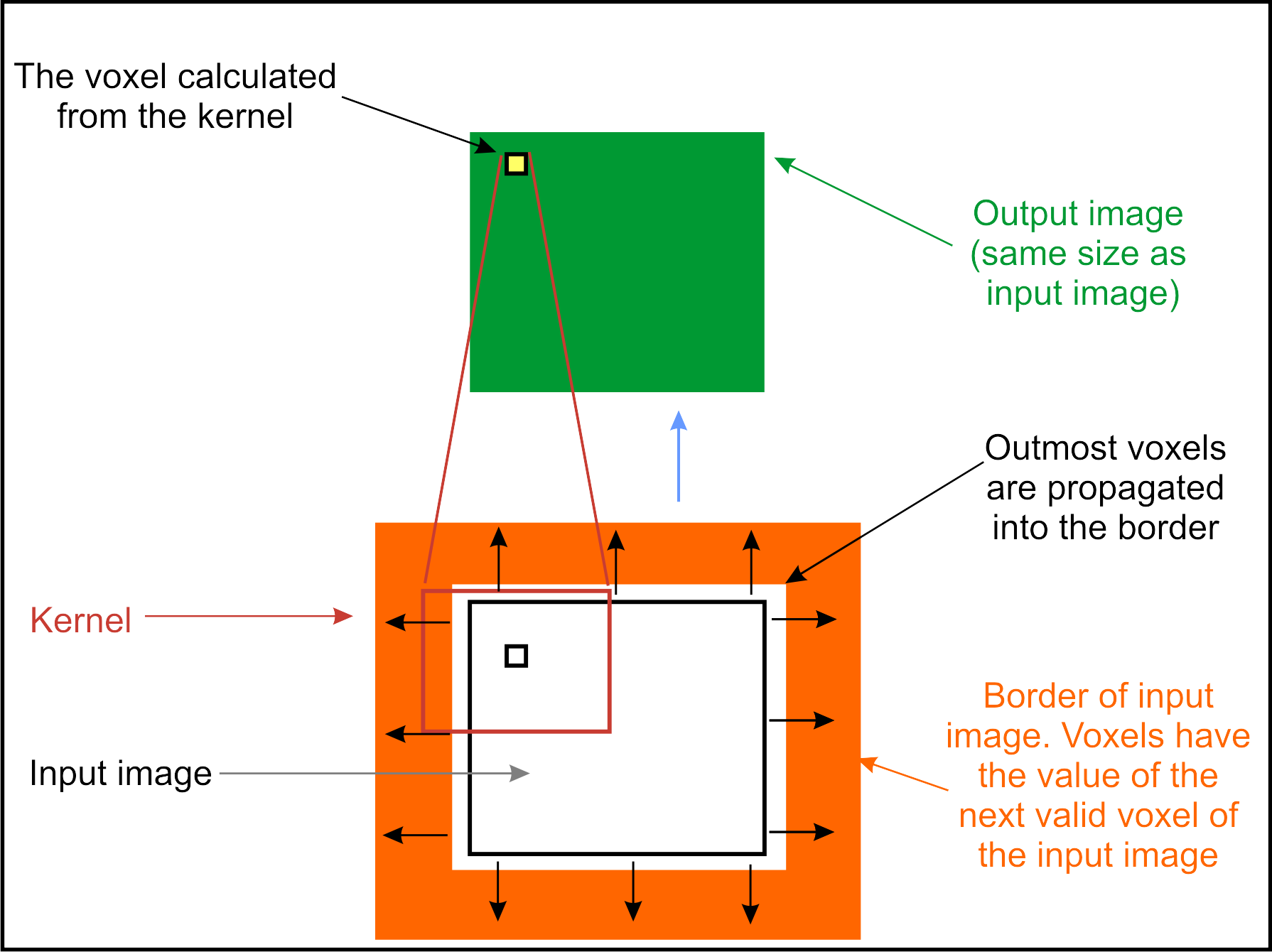NoteAnother mode `KernelTools::PAD_DST_CLAMP` would be desirable. It is not implemented since clamping to valid data does not work for requested pages which are completely outside the valid computable area. Slice 64, for instance, cannot be calculated if the last valid page is on slice 62 - and clamping to 62 cannot work, because 62 does not exist when a page in slice 64 is requested. It is theoretically possible to request it, but such a request would result in a very slow and difficult mode.

## 1.3. Kernel Classes

The following three sections give a detailed overview of the classes `KerneBaseModule,` `KernelModule` and `Kernel.`

Tool classes are described in Section 1.4, “ `KernelTools`.

### 1.3.1.  `KernelBaseModule`

This class adds some functionality to the `Module` class which is used by most kernel-based algorithms. This includes:

• a kernel (see Section 1.3.3, “ `Kernel`) member containing the structure element (kernel) for your algorithm, which can be accessed by the methods

• `getKernel()`

to access and modify the kernel object (read, set and modify kernel extent, elements, convert it from/to a string)

• `getConstKernel()`

to access and read the same kernel and its properties without the possibility to change it. Consequently, it can be called from constant methods.

• the method `getBorderHandlingFld()` which returns the field that manages the way how image borders are handled during kernel filtering. See Section 1.2, “Border Handling in Kernel Operations” for more information on these modes.

• the method `getFillValueFld()` which returns the field that manages a fill value used for image borders in some of the border handling modes. See Section 1.2, “Border Handling in Kernel Operations” for more information on these modes.

• an overloaded `calculateOutputImageProperties()` method which adapts the properties of the output image, i.e., it corrects the image extent and the voxel-to-world coordinate transformation. See Section 1.2, “Border Handling in Kernel Operations” for more information.

• an overloaded `calculateInputSubImageBox()` method which requests correct regions from the input image for the kernel filtering.

Although you will mainly derive your kernel-based ML module from the class `KernelModule`, you can also use the class `KernelBaseModule` without limitations. The class `KernelModule` adds much convenience functionality, which is often used in kernel-based modules, and therefore `KernelModule` is the recommended base class. See Section 1.3.2, “ `KernelModule` for detailed information.

In contrast to normal image processing modules, kernel-based modules use special template functions and macros:

```  CALC_ROW_CPP
CALC_ROW_CPP_ANY
CALC_ROW_CPP_EXT
CALC_ROW_CPP_EXT_DD
CALC_ROW_CPP_ANY_EXT

CALC_ROW_CPP_SUBIMG
CALC_ROW_CPP_ANY_SUBIMG
CALC_ROW_CPP_SUBIMG_EXT
CALC_ROW_CPP_SUBIMG_EXT_DD
CALC_ROW_CPP_ANY_SUBIMG_EXT```

In many kernel modules, using the `CALC_ROW_H()` and `CALC_ROW_CPP()` macros and a template function such as

```template <typename DATATYPE>
void StandardDeviationFilter::calcRow(MLsoffset    *indexTab,
size_t        indexTabSize,
MLsoffset     srcVoxelOffset,
size_t        numVox,
DATATYPE     *inCursor,
DATATYPE     *outCursor,
const ImageVector &rowStart)```

is sufficient as explained in Section 1.5, “Kernel Example for Page-Based Image Filtering”. They are used instead of the `ML_CALCULATE_OUTPUTSUBIMAGE_NUM_INPUTS_*` macros and the template function `calculateOutputSubImage`.

In the case of filtering with separable kernels, with more than one input or output, more flexible versions are needed. Use `CALC_ROW_H()` and `CALC_ROW_CPP_EXT()` macros and the following template function:

```    template <typename DATATYPE>
void SeparableKernelFilter::calcRow(MLsoffset    *indexTabs,
size_t        indexTabSize,
MLsoffset     srcVoxelOffset,
size_t        numVox,
DATATYPE    **inCursors,
DATATYPE     *outCursor,
const ImageVector &rowStart,
int           outIndex,
int           numInSubImgs,
size_t        separationPass,
size_t        iteration);```

There are derivates with similar function parameters using subimage parameters if subimage information is needed. Then the subimage cursors are set appropriately instead of the cursors:

```    template <typename DATATYPE>
void SeparableKernelFilter::calcRow(MLsoffset         *indexTabs,
size_t             indexTabSize,
MLsoffset          srcVoxelOffset,
size_t             numVox,
TSubImage<DATATYPE> *inSubImgs,
TSubImage<DATATYPE> &outSubImg,
const ImageVector &rowStart,
int                outIndex,
int                numInSubImgs,
size_t             separationPass,
size_t             iteration);```

All `CALC_ROW_*_EXT` versions also exist with _`DD` at the end to compile the `calcRow` template with different input and output types. Note that these versions compile considerable more code:

```    template <typename OT, typename IT>
void SeparableKernelFilter::calcRow(MLsoffset    *indexTabs,
size_t        indexTabSize,
MLsoffset     srcVoxelOffset,
size_t        numVox,
IT          **inCursors,
OT           *outCursor,
const ImageVector &rowStart,
int           outIndex,
int           numInSubImgs,
size_t        separationPass,
size_t        iteration);```

NoteFormer versions used `int` and `unsigned int` types instead of the now used `MLsoffset` and `size_t`. The `int` and `unsigned int` types do not change their size to 64 on 64 bit systems. In order to provide safe 64 bit operations, older code versions should be updated so that they use `MLsoffset` and `size_t` types.

See the `mlKernelMacros.h` for more information. The macros `_KERNEL_*_PARAMS` define the parameters of the template functions called by these macros.

Also see the examples `KernelExample`, `SeparableKernelExample` and `Kernel3In2OutExample` in project `MLKernelExamples` for details.

### 1.3.2.  `KernelModule`

The `KernelModule` class is quite a large convenience class derived from the `KernelBaseModule` class. It adds a lot of stuff needed in many kernel-based algorithms such as the following methods. See header file documentation for detailed information.

• A set of field creation methods normally called in the constructor for simple creation of the module parameter interface:

1. `virtual void _createKernelExtentFields(int numDim=6, const ImageVector &defaultExt=ImageVector(3,3,1,1,1,1))`

creates fields specifying the (6D) extent of the kernel.

2. `virtual void _createMakeSphericalField(bool defaultVal=false)`

creates an on/off parameter field which decides whether the kernel should be made roughly spherical. Sometimes "round" kernels are more suitable for filtering than rectangular ones.

3. `virtual void _createNormalizeField(bool defaultVal=false)`

creates an on/off parameter field which normalizes each kernel so that all kernel elements add up to 1.

4. `virtual void _createMinMaxCalcFields(bool defaultValue=false, bool createSetOutputMinMax=false)`

creates an on/off parameter field which adds a minimum/maximum value determination after filtering a page. The results can be used by the algorithm itself (e.g., to update the minimum/maximum values of the output image) or can be shown by the fields created by number 5

5. `virtual void _createMinMaxFields(MLdouble minVal=0, MLdouble maxVal=0, bool defaultValue=false)`

creates fields showing and updating the minimum and maximum values of the output image. See also number 4 for an automatic way of how to update these fields by using filtering results that have already been processed.

6. `virtual void _createExternalKernelFields(bool createUseToggle=false, bool defaultVal=false)`

creates a parameter field to which an external from another module can be connected as an "input connector" for the kernel. This way, the module can share its kernel with other modules in a network. See also number 7.

7. `virtual void _createKernelOutputField()`

creates a parameter field containing the kernel as a value which can be connected to other modules as an "output connector" for the kernel. This way, the internally used kernel can be shared with other modules in a network. See also number 6.

8. `virtual void _createImageIntervalFields(MLdouble min=0, MLdouble max=1024, bool createToggle=false, bool useIt=false)`

creates two parameter fields for an interval which can limit the number of voxels to be filtered. Thus filtering can easily be limited to the desired object which saves computing time and leaves other image areas unchanged or sets them to a certain value. See also number 1 to find out how your algorithm can check whether a voxel belongs to that interval.

9. `virtual void _createKernelIntervalFields(MLdouble min=0, MLdouble max=1024, bool createToggle=false, bool useIt=false)`

creates two or three fields for minimum and maximum interval limit and optionally as on/off toggle to activate or deactivate the interval. See also number 2 to find out how your algorithm can check whether a voxel belongs to that interval.

10. `virtual void _createKernelInfoField()`

creates a module (output) field showing some information about the kernel and its state.

11. `virtual void _createNumKernelElementsField()`

creates a module (output) field showing the number of elements in the kernel.

12. `virtual void _createKernelElementsSumField()`

creates a module (output) field showing the sum of all kernel elements.

• The following methods allow to check whether voxels are within an image or a kernel interval defined by number 8 or number 9:

1. `bool _isInImageInterval(MLdouble v) const`

to check whether the value `v` is within the interval specified by the fields number 8 created.

2. `bool _isInKernelInterval(MLdouble v) const`

to check whether the value `v` is within the interval specified by the fields number 9 created.

NoteThese intervals can be used to improve the speed and/or the quality of your filtering result: firstly, by reducing the amount of filtered voxels and secondly by explicitly selecting the voxels used in the filtering process itself which belong to the filtered object.
NoteNote that the intervals are considered being exclusive if maximum is smaller than minimum, i.e., all values between maximum and minimum are excluded and all other values are included. Intervals where minVal == maxVal are considered as ordinary intervals. Hence these intervals can easily be used to specify objects by voxels which are either inside or outside the interval.

• Derived modules often want to generate their own kernel before or after the kernel has been determined by the above fields. It can be useful to overload one of the following methods:

1. `virtual void _updateKernel(bool permitExtentChanges)`

is the core update method `KernelModule` uses to update the kernel to the state of the fields that have been created by the `create` methods described above, but also to update the kernel to the state of user-defined fields and settings. It also calls numbers 2 and 3 to enable derived classes to include their own kernel modifications.

2. `virtual void _userKernelPreUpdate()`

`_updateKernel(permitExtentChanges)` calls `virtual void _userKernelPreUpdate()` to supply a method where the derived class can change the kernel before the kernel input is checked or the kernel extent is set. See 1 for more information.

3. `virtual void _userKernelPostUpdate()`

`_updateKernel(permitExtentChanges)` calls `virtual void _userKernelPostUpdate()` to supply a method where the derived class can change the kernel after all automatic changes have been performed, and before the output kernel fields, the `_kernelInfoFld`, the `_numKernelElementsFld` and the `_kernelElementSumFld` are updated. See number 1 for more information.

• Some other helper routines are available in derived modules when they add their own kernel manipulations:

1. `virtual void _updateKernelOutputField()`

Updates the kernel output to the value of the kernel if the `_kernelOutputFld` is defined.

2. `virtual std::string _composeKernelInfo(bool valid=true) const`

Creates a string that contains all important kernel information. If `false` is passed, an "invalid" message is added.

These methods are normally called by 1; however, they may be useful when special kernel update algorithms are written.

• The following methods are also overloaded by `KernelModule` (see Section 1.3.2, “ `KernelModule` for more information), so be aware when overloading those methods: the superclass functionality must be called in derived modules to ensure correct operation. This is often not done in modules directly derived from `Module`, because the original `Module` methods are not used or are simply replaced by the derived classes.

1. `virtual void handleNotification(Field* field)`

2. `virtual void activateAttachments()`

3. `virtual void calculateOutputImageProperties(int outIndex)`

4. `virtual void calculateOutputSubImage(SubImage *outSubImg, int outIndex, SubImage *inSubImgs)`

### 1.3.3.  `Kernel`

The class `Kernel` provides a set of useful functionalities to support image processing algorithms using a structure element.

The following sections give an introduction to this class:

#### 1.3.3.1.  `Overview of the Kernel Class`

See the header file documentation of ``` mlKernel.h ``` in project `MLKernel`, for detailed information.

The file ``` mlKernel.h ``` contains the `TKernel` class to manage a 6D kernel which can be applied to images. It handles a set of coordinates (see `getCoordTab()`) and the corresponding values for these coordinates (see `getValueTab()`). This permits specifying kernels with gaps or with only a few defined elements. Thus big kernels with only a few elements (sparse kernels) can be quickly manipulated and applied.

The value and coordinate tables can be interpreted in two different ways: either as a structure element for a normal kernel filtering or as a separable kernel where the first six rows of the table are interpreted as the six 1D kernels which are used as kernels for separable filtering.

A set of operations can be performed on kernel instances, such as arithmetics on the kernel values, gauss presets, normalization, different kernel set/get routines to create/save partially defined kernels, get/set methods to load/save kernels as strings or arrays and much more. This `TKernel` class is implemented as template-dependent class on `KDATATYPE` to have different precisions for kernel elements. Normally, the kernel is instantiated with `double` as `KDATATYPE`, because it is the fastest type. You could also instantiate the `TKernel` class with `MLfloat` or `MLdouble`.

Note that using integer types for the kernels is not really useful since pure integer kernel operations are rare and some operations would suffer because of rounding errors or integer overflows that might occur when many kernel elements are summed up.

This class does not provide any support for applying the kernel to the image. However, `mlKernelTools` offers some helper functions for standard convolutions which can be called by the module directly and which can filter the (sub)images.

The kernel types that included `MLfloat` and `MLdouble` are predefined. Note that currently only the version with `MLdouble` is used in the kernel library.

```  typedef TKernel<MLfloat>        FloatKernel;
typedef TKernel<MLdouble>       DoubleKernel;
typedef MLdouble                KernelDataType;
typedef TKernel<KernelDataType> Kernel;```

#### 1.3.3.2.  `Basic Functionality of the Kernel Class`

The `TKernel` class provides a set of functions that offers the most important features required in image filtering algorithms.

• `TKernel();`

Constructor. Builds an empty kernel.

• `TKernel(const TKernel &kern);`

Copy constructor. Builds a kernel with contents of `kern`.

• `~TKernel();`

Destructor. Cleans up and removes instance of kernel.

• `const TKernel& operator=(const TKernel &kern);`

Assignment operator. Sets current instance to contents of `kern`.

• `void reset();`

Resets kernel to construction state.

• `unsigned int getTabSize() const;`

Returns the current number of kernel elements.

• `const ImageVector* getCoordTab(size_t dim = 0) const;`

Returns a table of coordinates pointing to all kernel elements that are currently defined. The size of the returned table is given by `getTabSize()`. In the case of separable filtering, it returns the coordinates that are valid for the current pass of separable kernel filtering. It is then a subset of the array given by `getSeparableCoordTab()`.

• `const KDATATYPE* getValueTab(size_t dim = 0) const;`

Returns the table of the kernel element values that are currently defined. The size of the returned table is given by `getTabSize()`. In the case of separable filtering, the subset of kernel elements for the pass `dim` can be requested.

• `KDATATYPE getValueTabSum() const;`

Returns the sum of all kernel element values.

• `KDATATYPE getMinValue() const;`

Returns the minimum value of all kernel element values. If the kernel value table is empty, 0 is returned.

• `KDATATYPE getMaxValue() const;`

Returns the maximum value of all kernel element values. If the kernel value table is empty, 1 is returned.

• `KDATATYPE getNegValueSum() const;`

Returns the sum of all negative kernel element values. If kernel the value table is empty, 0 is returned.

• `KDATATYPE getPosValueSum() const;`

Returns the sum of all positive kernel element values. If the kernel value table is empty, 0 is returned.

• `void manipulateKernelElements(KernModifier mode, KDATATYPE v);`

Modifies all kernel element values with the value `v`.

The `mode` can be one of the following:

- `KERN_SET `: Sets all kernel elements to `v`.

- `KERN_MULT` : Multiplies each kernel elements with `v`.

- `KERN_ADD` : Adds `v` to each kernel element.

- `KERN_INVDIV` : Each kernel element is replaced by `v` divided by the kernel element. Zero kernel elements are left unchanged.

- `KERN_INVSUB` : Each kernel element is replaced by `v` minus the kernel element.

- `KERN_SQR` : Each kernel element is replaced by its square.

- `KERN_SQRT` : Each kernel element is replaced by its square roots. Negative kernel elements are left unchanged.

- `KERN_POW` : Each kernel element is replaced by itself raised to the power of `v`.

- `KERN_LOG` : Each kernel element is replaced by its logarithm of `v.`

- `KERN_NORMALIZE` : Multiplies the all kernel element values with a value so that their sum is 1. If the sum is zero, values are left unchanged.

- `KERN_GAUSS` : Sets all kernel elements to binomial values and normalize.

- `KERN_SPHERE` : Throws the corners of the kernel value table away to make the kernel approximately spherical.

- `KERN_MIRROR `: Applies a point symmetric mirroring of all kernel elements.

- `KERN_MIRROR_X` : Applies a point symmetric mirroring of all kernel elements in x direction.

- `KERN_MIRROR_Y `: Applies a point symmetric mirroring of all kernel elements in y direction.

- `KERN_MIRROR_Z` : Applies a point symmetric mirroring of all kernel elements in z direction.

- `KERN_MIRROR_C` : Applies a point symmetric mirroring of all kernel elements in c direction.

- `KERN_MIRROR_T` : Applies a point symmetric mirroring of all kernel elements in t direction.

- `KERN_MIRROR_U `: Applies a point symmetric mirroring of all kernel elements in u direction.

• `const Vector &getExtent() const;`

Returns the kernel extent in 6D. It defines the rectangular region in which all coordinates returned by `getCoordTab()` are found. Note that the returned region might be larger than required e.g., after removing elements from the kernel. In the case of separable filtering, it returns a vector where the components [0...5] contain the extent of the region spanned by the 6 separated 1D kernels. For dimensions where no filtering takes place, the components are set to 1.

• `const ImageVector &getNegativeExtent() const;`

The extent of the kernel to both sides. The sum of both sides plus1 is the extent of the kernel. By using `getNegativeExtent()` as the negative extent of an image and `getPositiveExtent()` as the positive extent increment for the image, the kernel can be placed correctly on all normal image voxels without voxel accesses being out of range.

• `const ImageVector &getPositiveExtent() const;`

See `getNegativeExtent()`.

• `MLint coordToIndex(MLint x, MLint y, MLint z, MLint c, MLint t, MLint u, const ImageVector &size);`

Converts the coordinates `x, y, z, c, t and u` into the kernel to an index into an array with 6D extent given by `size`.

• `MLint coordToIndex(const ImageVector &p, const ImageVector &size);`

Converts the coordinate `p` into the kernel with extent `size` to an index.

• `ImageVector indexToCoord(MLint idx, const ImageVector &ext);`

Converts an index into an array with extent `ext` to a coordinate.

• `MLint findIndex(const ImageVector &pos) const;`

Returns the index to the kernel element if it exists; otherwise `-1` is returned. Note that this method needs to search, i.e., this method is not very efficient.

• `void setPartialKernel(size_t numElems, ImageVector * const coords, KDATATYPE * const values=NULL);`

Defines a set of local kernel coordinates and optionally defines a set of values that define the kernel elements. `numElems` defines the number of coordinates. If desired, the corresponding set of kernel values can be passed in `values`. Otherwise, all kernel values are set to `1.0/(KDATATYPE)_tabSize`. Note that `coords` and `values` have to contain `numElems` entries when passed.

• `std::string getKernel(bool asLines=false, MLint fieldWidth=0, MLint precision=10) const;`

Returns the current kernel elements and values as a string. The string must have the following format:

```    (x_0, y_0, z_0, c_0, t_0, u_0):v_0 \n
...\n
(x_n, y_n, z_n, c_n, t_n, u_n):v_n \n
```
where the coordinates to the left of the colon specify the 6D coordinate of the kernel element; the value `v_i` to the right of the colon specifies the value of the kernel element. If `asLines `is `true`, another string format is used:
```    (*, y_0, z_0, c_0, t_0, u_0):x_0 ... x_n \n
...\n
(*, y_n, z_n, c_n, t_n, u_n):y_n ... y_n \n
```
All kernel elements of a row are saved in a string line; the x coordinate becomes invalid and is replaced by an asterisk. To have a minimum field width, pass `fieldWidth` and the digits after the period if given by `precision`. Note that these settings are used only if `asLines` is `true`.

• `std::string setKernel(const std::string &kernString);`

Defines elements and values of the kernel matrix by parsing a string. The string must have the following format:

```    (x_0, y_0, z_0, c_0, t_0, u_0):v_0 \n
...\n
(x_n, y_n, z_n, c_n, t_n, u_n):v_n \n```
where the coordinates left to the colon specify the 6D coordinate of the kernel element; the value v_x right to the colon specify the value of the kernel element. Only one coordinate entry is scanned per line. Big kernels with a only a few elements can easily be set. As long as lines with kernel elements are found, elements are set in the kernel table. An empty string is returned for successful scans. For errors, the returned string contains the number of error lines. The following format is another way of how to specify more than one kernel element at once:

```    (*, y_0, z_0, c_0, t_0, u_0):x_0, ... ,x_n \n
...\n
(*, y_n, z_n, c_n, t_n, u_n):y_n, ... ,y_n \n```
All kernel elements of a row are found in a string line. Note that the x coordinate becomes invalid and must be replaced by an asterisk. Empty elements in the kernel can be left empty before, between and after commas.

• `void setKernel(const ImageVector &ext, const KDATATYPE * const values=NULL, bool * const mask=NULL);`

Defines a complete kernel matrix whose extent is defined by `ext`. If desired, the set of kernel values can be passed in `values`. Otherwise, all kernel values are to be set to `1.0/(KDATATYPE)ext.compMul()`, i.e., to the number of entries of the matrix. If desired, a set of mask values can be specified. If `mask[n]` is `true`, `tab[n]` is included in the kernel; otherwise, it is not part of the kernel. The specified kernel matrix is internally handled as a table of kernel elements. Note that `values` and `mask` must have `ext.compMul()` elements if they are defined.

• `template <typename KDATATYPE2> `

`void setKernel(const ImageVector &ext, `

` const KDATATYPE2 * const xaxis, `

` const KDATATYPE2 * const yaxis, `

` const KDATATYPE2 * const zaxis, `

` const KDATATYPE2 * const caxis, `

` const KDATATYPE2 * const taxis, `

` const KDATATYPE2 * const uaxis, `

` bool normalize)`

Defines a complete kernel matrix whose extent is defined by `ext`. The values of the kernel elements are products of the corresponding values along the six axes given as parameters. This is a convenient way to build a kernel from separated axis. If desired, the kernel is normalized.

• `void setSeparableKernel(const std::vector<ML_TYPENAME std::vector<KDATATYPE> > &separableRows);`

Creates kernel coordinate and value tables in a separable table format, that means a 2D kernel, in which each row describes the elements of 1D kernels. The number of rows should not exceed 6, because the maximum kernel dimension is 6. It is legal to pass empty vectors in order to ignore axes and thus to define separable kernels with less than 6 dimensions. The separability flag is set to `true`.

• `void resizeKernel(const ImageVector &ext, KDATATYPE newVal=0);`

Resizes the kernel to a new state and tries to maintain the previous elements if possible. Note that the new kernel size may differ from desired kernel size if empty areas are on the border of the resized kernel so that only a smaller actual kernel remains. Newly created regions receive kernel elements with value `newVal`.

• `void fillUndefinedElements(KDATATYPE newVal=0);`

Fill all undefined kernel element with `newVal`.

• `void makeCircular();`

Takes the current kernel, computes radii from the extent of the kernel and removes all kernel elements which are outside the ellipsoid defined by these radii. Note that this operation changes the kernel filter's properties.

• `void mirror(int dimension=-1);`

Applies point-symmetric mirroring to all kernel elements. The `dimension` specifies a mirroring dimension if in [0...5], otherwise, mirroring is applied in all dimensions. The default is -1.

• `static MLldouble binomialcoeff(MLint n, MLint k);`

Calculates binomial coefficients for (n) over (k).

• `static std::vector<KDATATYPE> get1DGauss(size_t numSamples, bool normalize=true);`

Returns a vector with `numSample` values binomial coefficients. If `numSamples` is passed as 0, an empty vector is returned. If `normalize` is passed as `true` (the default), all values are normalized to the sum of absolute values 1.

• `void setGauss(const ImageVector &ext);`

Replaces the current kernel by a normalized gauss kernel.

#### 1.3.3.3.  `Support for an Interpretation as Separable Kernel`

If the kernel is interpreted as a separable kernel, the first six rows of the first slice of the kernel matrix are taken. These six rows are interpreted as the six 1-dimensional axes in x,y,z,c,t, and u dimensions which are used as 1D filter kernels for 6D separable filtering.

Be sure to use a `CALC_ROW_*_EXT` macro instead of a `CALC_ROW_*` macro in your module implementation so that you have all the necessary parameters for your `calcRow` template function when you implement separable filtering.

For example:

```  | 1 3 5 6 4 |
| 2 7 2     |
| 4 1 9 5   |
|           |
| 3 8 3     |
|           |```

describes a separable kernel with the following axes extent: X=5, Y=3, Z=4, C=0, T=3, U=0. An extent of 0 (C and U dimensions, for example) is used to define that filtering with a 1D kernel is not applied in that dimension.

• `void setSeparable(bool isSeparable);`

Sets a flag to indicate that the first 6 rows of the first kernel slice are considered as the 1D axes of a separable filter kernel. Note that this flag only defines how applications shall interpret this kernel; the flag does not change any behavior of this class.

• `bool isSeparable() const;`

Indicates whether the first 6 rows of the first kernel slice are interpreted as 1D axes of a separable filter kernel; the default is `false`.

• `ImageVector getSeparableDimEntries() const;`

Returns a vector with the number of entries of the separable kernel for dimensions [0...5]. This means the ith index contains the number of `valueTab` entries of row i, where i is in [0...5].

• `ImageVector getSeparableOneDimExtents() const;`

Returns a vector where the components [0...5] contain the extent of the region spanned by the 6 separated 1D kernels if the kernel is interpreted as a separable kernel. Note that components of the extent are 0 for those dimensions where no entries are available.

• `size_t getSeparableDimIndex(size_t dim=0) const;`

Returns the index to entries of `valueTab` or `coordTab `which are related to the 1D separable kernel for the dimension `dim` where `dim` must be in [0...5]. Smaller values are clamped to 0, higher values are clamped to 5. The index to the entries for the 1D kernel of the x-dimension is returned with the default parameter.

• `const std::vector<ImageVector> &getSeparableCoordTab() const;`

Returns the table with all coordinates for filtering with separable kernels.

## 1.4.  `KernelTools`

The class `KernelTools` can considerably simplify kernel filtering of images . Many of the functions are used by the `KernelBaseModule` and `KernelModule`, but some functions are useful for normal implementations of kernel filters:

• the `BorderHandling` enum type and a set of string names that correspond to the enum values.

• `createIndexTab`: Creates the offset table from a pointer to a kernel origin to the image voxels below kernel elements.

• `calcSrcVoxelOffset`: Calculates the offset from a pointer to a kernel origin to that voxel in the input image (below kernel) corresponding to the written output voxel.

• `calcOutImageExt`: Calculates the extent of an output image from the kernel extent and the input image extent and the border handling mode. Useful in `calculateOutputImageProperties()`.

• `adaptWorldCoordinates`: Corrects the world coordinates of a kernel-filtered output image dependent on the border handling mode. Useful in `calculateOutputImageProperties()`. Usually already done in kernel base classes.

• `calcInSubImageBoxForOutSubImg`: Calculates the region of the input image needed to calculate a kernel-filtered region of the output image. Useful in `calculateInputSubImageBox()`. Usually already done by kernel base classes.

• `calcOutInCoordShift`: Calculates the voxel shift between input image and output image of a kernel-filtered image dependent on kernel extent and border handling mode.

• `calcAreaToBeCalculated`: Calculates the entire region of the output image which needs to be calculated/written by kernel filtering.

• `copyLine`: Copies the row to be filtered from the input buffer to the output buffer.

• `correlateLine`: Correlates the row to be filtered with the current settings.

• `correlateLineWithImageInterval`: Correlates all voxels in the row to be filtered if they are within a certain interval. All other voxels are simply copied.

• `correlateLineWithKernelInterval`: Correlates a row of the input image and writes the result to the corresponding row of the output image. Voxels which are not part of a kernel interval are not included in the correlation process.

• `correlateLineWithImageAndKernelInterval`: Correlates a row of the input image and writes the result to the corresponding row of the output image. Voxels which are not in a kernel interval are not included in the correlation process. Voxels that are not in an image interval are simply copied from the input image to the output image without being filtered.

• `fillBorders`: Fill borders of an input or output subimage correctly dependent on the border handling mode and the image extent.

• `applyFiltering`: Two versions of this function allow to apply the filtering function to a subimage and to write the result to an output image dependent on border handling and fill values.

Tip`correlateLine` implements a standard correlation. `correlateLineWithImageInterval` is a powerful alternative which only filters a subset of image voxels specified by an interval. That can save a lot of computing time. These functions might be useful in many kernel filter implementations, and `KernelModule` already supports their parameters.
NoteThe `KernelTools` provide functionality to correlate an image with a kernel. For convolution, the kernel must be point-mirrored at its center. Thus, correlation is identical with convolution for point-symmetric kernels.

## 1.5. Kernel Example for Page-Based Image Filtering

This section gives an example of how to implement a kernel filter where a gauss kernel is used to filter the input image.

This module also uses some base class functionality.

• selectable border handling mode (from `KernelBaseModule`)

• fill value for undefined areas in image borders (from `KernelBaseModule`)

• an image interval which limits the number of filtered voxels to those voxels that are within that interval (from `KernelModule`)

• a kernel input field where a kernel from another module can be connected which is then used for filtering (from `KernelModule`)

It also uses functionality from the class `KernelTools` which applies a kernel to a row of image voxels in a subimage.

Example 1.1. How to Implement a Kernel-Based Module

```#pragma once

#include <mlModuleIncludes.h>
#include "mlKernel.h"
#include "mlKernelModule.h"
```

```ML_START_NAMESPACE

//--------------------------------------------------
//! The class to convolute an image.
//--------------------------------------------------
class ML_EXAMPLE_CONVOLOUTION_EXPORT ExampleConvolutionFilter
: public KernelModule
{

public:
//! Constructor. Initializes the fields, the members and the field
//! connections with the field container.
ExampleConvolutionFilter();

```
```  protected:
//! Called when a parameter field is changed.

//! Updates the kernel to new field state and sets the new
//! kernel for filtering.
virtual void _updateKernel();

//! Computes the output image properties from the input image
//! properties and the _convKernelFld.
virtual void calculateOutputImageProperties(int outIndex);

//! The implementation of the calculateOutputSubImage class overloaded from
//! Module is done in this macro. It implements page border handling
//! and a dispatcher to call the correct template version of calcRow
//! with the correct parameters.
//! Note that CALC_ROW_CPP() also needs to be added in the .cpp file.
CALC_ROW_H();```
```    //! In this virtual template method the filtering of
//! one row needs to be implemented.
//! It will be called by the CALC_ROW_H / CALC_ROW_CPP macro.
template <typename DATATYPE>
void calcRow(MLsoffset    *indexTab,
size_t        indexTabSize,
MLsoffset     srcVoxelOffset,
size_t        numVox,
DATATYPE     *inCursor,
DATATYPE     *outCursor,
const ImageVector &/*rowStart*/);

//! Macro to declare methods/functions of the runtime system interface of
//! this class. It is defined in mlRuntimeSubClass.h. Note that this
//! class must be registered in the project initialization file by calling
//! the initClass() function implemented in this macro.
};

ML_END_NAMESPACE```

The source code:

```#include "mlMLGuideExampleConvolutionFilter.h"
#include "mlKernelTools.h"

ML_START_NAMESPACE

//---------------------------------------------------------------------------
// Macro to implement runtime type system functions (see mlRuntimeSubClass.h)
//---------------------------------------------------------------------------
ML_MODULE_CLASS_SOURCE_EXT(ExampleConvolutionFilter,
KernelModule, :KernelModule())```
```  //---------------------------------------------------------------------------
//! Constructor: Activate/Add (base) class fields and initialize kernel.
//---------------------------------------------------------------------------
ExampleConvolutionFilter::ExampleConvolutionFilter() : KernelModule()
{
// Set a flag that all fields are still invalid.

// Activate inherited fields: See KernelModule.h
_createImageIntervalFields(0, 1024, true, false);
_createExternalKernelFields(true, false);
_createKernelInfoField();
_createKernelOutputField();

// Now all fields are okay and their changes can be handled

// Set kernel corresponding to the current settings.
_updateKernel();
}  ```
```  //---------------------------------------------------------------------------
//! Called when a field in the field container is changed.
//---------------------------------------------------------------------------
{
// Check your own field changes (not in this example)
//if (field == _myOwnFld){ _updateKernel(); }

// Call superclass stuff.
}```
```  //---------------------------------------------------------------------------
// update the kernel to the new field state.
//---------------------------------------------------------------------------
void ExampleConvolutionFilter::_updateKernel()
{
// Define a filter kernel and its extent. The kernel
// is symmetric, so the correlation
// is the same as a convolution.
ImageVector ext55(5,5,1,1,1,1);

// Unnormalized 5 x 5 Gauss Kernel
KernelDataType Kerneldata55Gauss[]={1,  4,  6,  4, 1,
4, 16, 24, 16, 4,
6, 24, 36, 24, 6,
4, 16, 24, 16, 4,
1,  4,  6,  4, 1};

// Get kernel from base class, set it to upperly defined
// kernel matrix and normalize it.
getKernel().setKernel(ext55, Kerneldata55Gauss);
getKernel().manipulateKernelElements(Kernel::KERN_NORMALIZE, 0);

// Kernel connected to kernel input field (which is
// inherited from KernelModule)?
if (getUseExternalKernelFld()->getBoolValue()){
// Yes, user has selected to use the kernel from the external
// kernel field. Permit extent
// changes (i.e., input kernel setting) in superclass call.
KernelModule::_updateKernel(true);
}
else{
// Input is NOT used. Forbid extent changes (i.e., input kernel
// setting) in superclass.
KernelModule::_updateKernel(false);
}
}```
```  //---------------------------------------------------------------------------
//! Computes the output image properties from the input image properties.
//---------------------------------------------------------------------------
void ExampleConvolutionFilter::calculateOutputImageProperties(int outIndex, PagedImage* outputImage)
{
// For normal convolutions/correlations use convenience method to
// determine new min/max values.
_setCorrectCorrelationMinMax(
getUseImageIntervalFld() && getUseImageIntervalFld()->isOn(),
getImageIntervalMinFld() ? getImageIntervalMinFld()->getDoubleValue() : 0,
getImageIntervalMaxFld() ? getImageIntervalMaxFld()->getDoubleValue() : 0);

// Execute superclass stuff.
KernelModule::calculateOutputImageProperties(outIndex, outputImage);
}```
```  //---------------------------------------------------------------------------
//! Macro which needs to be added to the implementation
//! of a class dervied from KernelModule or
//! KernelBaseModule. It guarantees that the correct
//! template functions are called for images
//! with any data type. It also overloads calculateOutputSubImage
//! of the base class Module.
//---------------------------------------------------------------------------
CALC_ROW_CPP(ExampleConvolutionFilter, KernelModule);```
```  //---------------------------------------------------------------------------
//! Calculates the result page in the desired data type.
//! Called by calculateOutputSubImage
//! which is implemented as a macro in .cpp.
//---------------------------------------------------------------------------
template <typename DATATYPE>
void ExampleConvolutionFilter::calcRow(MLsoffset    *indexTab,
size_t        indexTabSize,
MLsoffset     srcVoxelOffset,
size_t        numVox,
DATATYPE     *inCursor,
DATATYPE     *outCursor,
const ImageVector &/*rowStart*/)
{
if (getUseImageIntervalFld() && getUseImageIntervalFld()->isOn()){
// Convolute/correlate all row voxels with obey the image interval.
KernelTools::correlateLineWithImageInterval(inCursor, outCursor, numVox,
getKernel().getValueTab(),
indexTab, indexTabSize, srcVoxelOffset,
getImageIntervalMinFld()->getDoubleValue(),
getImageIntervalMaxFld()->getDoubleValue());
}
else{
// Correlate/Convolute the line normally.
KernelTools::correlateLine(inCursor, outCursor, numVox,
getKernel().getValueTab(),
indexTab, indexTabSize);
}
}

ML_END_NAMESPACE```

In case you want to implement your own filter instead of using functionality from the class `KernelTools` you can have a look at the following example implementing a very simple sum filter. It sums up all values under kernel elements and then adds the kernel elements.

```  //---------------------------------------------------------------------------
//! Calculates the result page in the desired data type.
//! Called by calculateOutputSubImage
//! which is implemented in CALC_ROW_CPP(...).
//---------------------------------------------------------------------------
template <typename DATATYPE>
void SumUpFilter::calcRow(MLsoffset    *indexTab,
size_t       indexTabSize,
MLsoffset    srcVoxelOffset,
size_t       numVox,
DATATYPE     *inCursor,
DATATYPE     *outCursor,
const ImageVector &rowStart)
{
// Get pointer to first value of kernel element table.
KernelDataType *kElementVals = getKernel().getValueTab();

// Process all voxels in subimage row whose start is given by inCursor.
for (size_t i=0; i < numVox; i++){
// Get the voxel value under the input image voxel to be
// replaced in output image (not needed here):
// DATATYPE srcVox = inCursor[srcVoxelOffset];

// Sum up input image voxels under all kernel
// elements and add kernel element values.
// Input image voxels can be addressed by offsetting
// the pointer to the input image
// voxel with the indices from indexTab.
for (size_t c = 0; c < indexTabSize; ++c){
*outCursor += inCursor[indexTab[c]] + kElementVals[c];
}

// Move read pointer (into input subimage) and write cursor
// into output page) forward.
++outCursor;
++inCursor;
}
}```

## 1.6. Traps and Pitfalls in Kernel Programming

This sections discusses typical errors when programming kernel-based filters.

Typical errors are

• to forget to call superclass functionality in `handleNotification`, `activateAttachments` and the various `calc*` methods.

In classes directly derived from `Module,` this is usually not necessary, because the `Module` methods are empty or are simply replaced. `KernelBaseModule` and `KernelModule`, however, implement important functionality which is needed for correct operation.

• to change the kernel value at the wrong position in the class when you derive from `KernelModule`. This can lead to invalid or no filtering of image data.

Refer to `_userKernelPreUpdate()`, `_userKernelPostUpdate()`, and `_updateKernel()` documentation for detailed information on how the change the kernel value at the correct position.

• to implement the numerical part of kernel filtering with the template data type without considering that the data type may also be an integer type with limited precision.

Refer to the comments at the end of the class `KernelExample` and to Compile and Runtime Decisions on Standard and Registered Voxel Types for detailed information on how to implement different algorithms for floating point, integer or registered voxel types.

• to forget to add newlines to the end of lines of copied kernel strings e.g., from the `KernelEditor` module. When using the `setKernel(std::string)` method of the `Kernel` class, all element description lines must be separated by "\n":

``` getKernel().setKernel("(*,0,0,0,0,0):  0.06,  0.13,  0.06 \n"
"(*,1,0,0,0,0):  0.13,  0.25,  0.13 \n"
"(*,2,0,0,0,0):  0.06,  0.13,  0.06 \n");```